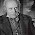## Sunday, July 5, 2015

### More on causes of death in Netherlands over the years

Last week I had a post 'Deaths in the Netherlands by cause and age'. During creation of that post I made one plot which I had not shown. It shows something odd. There is a vertical striping. Hence mortality varies by year across age.
To examine this phenomenon further here is a plot of some underlying causes. I would say the striping is present for at least three categories; "Diseases of the circulatory system", "Diseases of the respiratory organs" and "Sympt., Abnormal clinical Observations". This is odd, since these do not seem to be contagious. I suspect therefore that something like harsh weather (heat or cold) makes life more difficult, but does not get to be the final cause in the administration.
In addition there is something which I did not realize before regarding "Mental and behavioral disorders". They are age related. But it also seems that somewhere in the nineties of last century they became acceptable to register. And suddenly they are present, across several age categories.
This plot, same data, differently organized, shows that the years with these causes are similar, especially "Diseases of the circulatory system" and "Diseases of the respiratory organs"

### Can it statistically be seen?

It is very nice that I can see that, but how about measuring it? Hence for age 90 to 95, after detrending, correlation between the two most visually correlated causes of death.
Pearson's product-moment correlation

data:  xx\$`Diseases of the respiratory organs` and xx\$`Diseases of the circulatory system`
t = 2.4997, df = 62, p-value = 0.01509
alternative hypothesis: true correlation is not equal to 0
95 percent confidence interval:
0.06133681 0.51042863
sample estimates:
cor
0.302584

### Code

library(dplyr)
library(ggplot2)
txtlines <- grep('Centraal',txtlines,value=TRUE,invert=TRUE)
#txtlines[1:5]
#cat(txtlines)
col.names=c('Causes','Causes2','Age','year','aantal','count'),
na.strings='-',text=txtlines[3:length(txtlines)]) %>%
select(.,-aantal,-Causes2)
transcauses <- c(
"Infectious and parasitic diseases",
"Diseases of skin and subcutaneous",
"Diseases musculoskeletal system and connective ",
"Diseases of the genitourinary system",
"Pregnancy, childbirth",
"Conditions of perinatal period",
"Congenital abnormalities",
"Sympt., Abnormal clinical Observations",
"External causes of death",
"Neoplasms",
"Illness of blood, blood-forming organs",
"Endocrine, nutritional, metabolic illness",
"Mental and behavioral disorders",
"Diseases of the nervous system and sense organs",
"Diseases of the circulatory system",
"Diseases of the respiratory organs",
"Diseases of the digestive organs",
"Population",
"Total all causes of death")
#cc <-
cbind(transcauses,levels(r1\$Causes))
options(width=100)
levels(r1\$Causes) <- transcauses
levels(r1\$Age) <-
gsub('jaar','year',levels(r1\$Age)) %>%
gsub('tot','to',.) %>%
gsub('of ouder','+',.)
r1 <- mutate(r1,age=as.numeric(sub(' .*\$','',Age)))

Deaths <- filter(r1,Causes=='Total all causes of death') %>%
mutate(.,Total=count) %>%
select(.,-count,-Causes) %>%
merge(.,r1) %>%
filter(.,Causes %in% transcauses) %>%
mutate(.,Population=count,
Percentage=100*Total/Population,
year = as.numeric(gsub('*','',year,fixed=TRUE))) %>%
select(.,-Causes,-count)

png('deathall.png')
v <- ggplot(Deaths[Deaths\$age>60,], aes( year,Age, fill = Percentage))
v + geom_raster() +
colours=c('white','black'))+
theme(legend.position="bottom")+
ggtitle('Total all causes of death')
dev.off()

v3 <- filter(r1,Causes %in% transcauses,
age>65) %>%
mutate(.,Population=count) %>%
select(.,-count,-Causes) %>%
merge(.,r1) %>%
filter(.,Causes %in% transcauses[c(8,15,9,10,13,16)]) %>%
mutate(.,Total=count,
Percentage=100*Total/Population,
year = as.numeric(gsub('*','',year,fixed=TRUE))) %>%
select(.,-count)
png('bycause.png')
ggplot(v3, aes( year,Age, fill = Percentage))+
geom_raster() +
colours=c('white','black'))+
theme(legend.position="bottom")+
facet_wrap( ~ Causes,nrow=3)
dev.off()

png('byage.png')
ggplot(v3[v3\$age>75,], aes( year,Causes, fill = Percentage))+
geom_raster() +
colours=c('white','black'))+
theme(legend.position="bottom")+
facet_wrap( ~ Age,nrow=3)
dev.off()

xx <- filter(r1,Causes %in% transcauses,
age==90) %>%
mutate(.,Population=count) %>%
select(.,-count,-Causes) %>%
merge(.,r1) %>%
filter(.,Causes %in% transcauses[c(8,15,9,16)]) %>%
mutate(.,Total=count,
Percentage=100*Total/Population,
year = as.numeric(gsub('*','',year,fixed=TRUE)),
Causes=factor(Causes)) %>%
select(.,-count,-Age,-age,-Population,-Total) %>%
reshape(.,direction='wide',timevar='Causes',idvar='year')

names(xx) <- gsub('Percentage.','',names(xx))
for (i in 2:ncol(xx)) xx[,i]<- xx[,i] - predict(loess(xx[,i] ~ year,data=xx))

cor.test(xx\$`Diseases of the respiratory organs`,
xx\$`Diseases of the circulatory system`)

#### 1 comment:

1.Very interesting. Assuming I understand what you mean by vertical stripping I would say that, given your time scale, many of the strips are too wide to be accounted for by seasonal variation. Seems like something else is going--an artifact perhaps? Several look to me to be at least a year wide. Also, the is correlation value 0.302584 appreciable for this kind of data? I know that with some kinds of socio-metric data correlations of 0.3 ~ 0.5 are sometimes considered to be significant even though in other areas the would not be. As a physical scientist I would expect correlations much closer to 1.